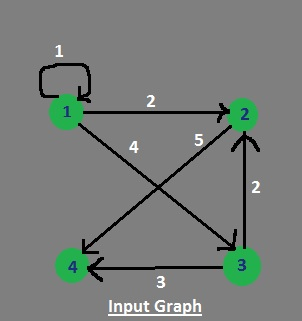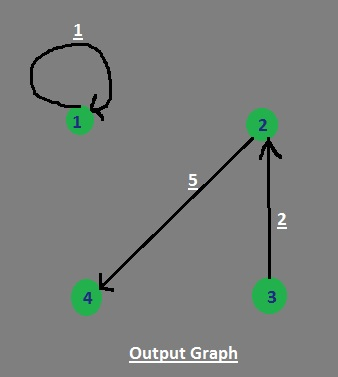# Remove all outgoing edges except edge with minimum weight

Given a directed graph having n nodes. For each node, delete all the outgoing edges except the outgoing edge with minimum weight. Apply this deletion operation for every node and then print the final graph remained where each node of the graph has at most one outgoing edge and that too with minimum weight.

Note: Here, graph is stored as Adjacency Matrix for ease.

Examples :

```Input : Adjacency Matrix of input graph :
| 1  2  3  4
---------------
1 | 0  3  2  5
2 | 0  2  4  7
3 | 1  2  0  3
4 | 5  2  1  3

Output : Adjacency Matrix of output graph :
| 1  2  3  4
---------------
1 | 0  0  2  0
2 | 0  2  0  0
3 | 1  0  0  0
4 | 0  0  1  0
```

## Recommended: Please try your approach on {IDE} first, before moving on to the solution.

For every row of the adjacency matrix of graph keep the minimum element (except zero) and make rest of all zero. Do this for every row of the input matrix. Finally, print the resultant Matrix.

Example :## C++

 `// CPP program for minimizing graph ` `#include ` ` `  `using` `namespace` `std; ` ` `  `// Utility function for ` `// finding min of a row ` `int` `minFn(``int` `arr[]) ` `{ ` `    ``int` `min = INT_MAX; ` ` `  `    ``for` `(``int` `i = 0; i < 4; i++) ` `        ``if` `(min > arr[i]) ` `            ``min = arr[i]; ` `    ``return` `min; ` `} ` ` `  `// Utility function for minimizing graph ` `void` `minimizeGraph(``int` `arr[]) ` `{ ` `    ``int` `min; ` ` `  `    ``// Set empty edges to INT_MAX ` `    ``for` `(``int` `i = 0; i < 4; i++) ` `        ``for` `(``int` `j = 0; j < 4; j++) ` `            ``if` `(arr[i][j] == 0) ` `                ``arr[i][j] = INT_MAX; ` ` `  `    ``// Finding minimum of each row ` `    ``// and deleting rest of edges ` `    ``for` `(``int` `i = 0; i < 4; i++) { ` ` `  `        ``// Find minimum element of row ` `        ``min = minFn(arr[i]); ` ` `  `        ``for` `(``int` `j = 0; j < 4; j++) { ` `            ``// If edge value is not min ` `            ``// set it to zero, also ` `            ``// edge value INT_MAX denotes that ` `            ``// initially edge value was zero ` `            ``if` `(!(arr[i][j] == min) || (arr[i][j] == INT_MAX)) ` `                ``arr[i][j] = 0; ` `            ``else` `                ``min = 0; ` `        ``} ` `    ``} ` ` `  `    ``// Print result; ` `    ``for` `(``int` `i = 0; i < 4; i++) { ` `        ``for` `(``int` `j = 0; j < 4; j++) ` `            ``cout << arr[i][j] << ``" "``; ` `        ``cout << ``"\n"``; ` `    ``} ` `} ` ` `  `// Driver Program ` `int` `main() ` `{ ` `    ``// Input Graph ` `    ``int` `arr = { 1, 2, 4, 0, ` `                      ``0, 0, 0, 5, ` `                      ``0, 2, 0, 3, ` `                      ``0, 0, 0, 0 }; ` ` `  `    ``minimizeGraph(arr); ` ` `  `    ``return` `0; ` `} `

## Java

 `// Java program for ` `// minimizing graph ` `import` `java.io.*; ` `import` `java.util.*; ` `import` `java.lang.*; ` ` `  `class` `GFG { ` ` `  `    ``// Utility function for ` `    ``// finding min of a row ` `    ``static` `int` `minFn(``int` `arr[]) ` `    ``{ ` `        ``int` `min = Integer.MAX_VALUE; ` ` `  `        ``for` `(``int` `i = ``0``; ` `             ``i < arr.length; i++) ` `            ``if` `(min > arr[i]) ` `                ``min = arr[i]; ` `        ``return` `min; ` `    ``} ` ` `  `    ``// Utility function ` `    ``// for minimizing graph ` `    ``static` `void` `minimizeGraph(``int` `arr[][]) ` `    ``{ ` `        ``int` `min; ` ` `  `        ``// Set empty edges ` `        ``// to INT_MAX ` `        ``for` `(``int` `i = ``0``; ` `             ``i < arr.length; i++) ` `            ``for` `(``int` `j = ``0``; ` `                 ``j < arr.length; j++) ` `                ``if` `(arr[i][j] == ``0``) ` `                    ``arr[i][j] = Integer.MAX_VALUE; ` ` `  `        ``// Finding minimum of each ` `        ``// row and deleting rest ` `        ``// of edges ` `        ``for` `(``int` `i = ``0``; ` `             ``i < arr.length; i++) { ` ` `  `            ``// Find minimum ` `            ``// element of row ` `            ``min = minFn(arr[i]); ` ` `  `            ``for` `(``int` `j = ``0``; ` `                 ``j < arr.length; j++) { ` `                ``// If edge value is not ` `                ``// min set it to zero, ` `                ``// also edge value INT_MAX ` `                ``// denotes that initially ` `                ``// edge value was zero ` `                ``if` `((arr[i][j] != min) || (arr[i][j] == Integer.MAX_VALUE)) ` `                    ``arr[i][j] = ``0``; ` `                ``else` `                    ``min = ``0``; ` `            ``} ` `        ``} ` ` `  `        ``// Print result; ` `        ``for` `(``int` `i = ``0``; ` `             ``i < arr.length; i++) { ` `            ``for` `(``int` `j = ``0``; ` `                 ``j < arr.length; j++) ` `                ``System.out.print(arr[i][j] + ``" "``); ` `            ``System.out.print(``"\n"``); ` `        ``} ` `    ``} ` ` `  `    ``// Driver Code ` `    ``public` `static` `void` `main(String[] args) ` `    ``{ ` `        ``// Input Graph ` `        ``int` `arr[][] = { { ``1``, ``2``, ``4``, ``0` `}, ` `                        ``{ ``0``, ``0``, ``0``, ``5` `}, ` `                        ``{ ``0``, ``2``, ``0``, ``3` `}, ` `                        ``{ ``0``, ``0``, ``0``, ``0` `} }; ` ` `  `        ``minimizeGraph(arr); ` `    ``} ` `} `

## Python3

 `# Python3 program for minimizing graph  ` ` `  `# Utility function for finding min of a row  ` `def` `minFn(arr):  ` ` `  `    ``minimum ``=` `float``(``'inf'``)  ` `     `  `    ``for` `i ``in` `range``(``0``, ``4``):  ` `        ``if` `minimum > arr[i]:  ` `            ``minimum ``=` `arr[i]  ` `    ``return` `minimum ` ` `  `# Utility function for minimizing graph  ` `def` `minimizeGraph(arr):  ` ` `  `    ``# Set empty edges to INT_MAX  ` `    ``for` `i ``in` `range``(``0``, ``4``):  ` `        ``for` `j ``in` `range``(``0``, ``4``):  ` `            ``if` `arr[i][j] ``=``=` `0``:  ` `                ``arr[i][j] ``=` `float``(``'inf'``)  ` ` `  `    ``# Finding minimum of each row  ` `    ``# and deleting rest of edges  ` `    ``for` `i ``in` `range``(``0``, ``4``):  ` `         `  `        ``# Find minimum element of row  ` `        ``minimum ``=` `minFn(arr[i])  ` `         `  `        ``for` `j ``in` `range``(``0``, ``4``): ` `             `  `            ``# If edge value is not min  ` `            ``# set it to zero, also  ` `            ``# edge value INT_MAX denotes that  ` `            ``# initially edge value was zero  ` `            ``if` `((``not``(arr[i][j] ``=``=` `minimum)) ``or`  `                    ``(arr[i][j] ``=``=` `float``(``'inf'``))):  ` `                ``arr[i][j] ``=` `0` `            ``else``: ` `                ``minimum ``=` `0` `         `  `    ``# Print result  ` `    ``for` `i ``in` `range``(``0``, ``4``):  ` `        ``for` `j ``in` `range``(``0``, ``4``):  ` `            ``print``(arr[i][j], end ``=` `" "``)  ` `        ``print``()  ` ` `  `# Driver Code  ` `if` `__name__ ``=``=` `"__main__"``:  ` `     `  `    ``# Input Graph  ` `    ``arr ``=` `[[``1``, ``2``, ``4``, ``0``],  ` `           ``[``0``, ``0``, ``0``, ``5``],  ` `           ``[``0``, ``2``, ``0``, ``3``],  ` `           ``[``0``, ``0``, ``0``, ``0``]]  ` `     `  `    ``minimizeGraph(arr)  ` `     `  `# This code is contributed by  ` `# Rituraj Jain `

## C#

 `// C# program for ` `// minimizing graph ` `using` `System; ` ` `  `class` `GFG { ` ` `  `    ``// Utility function for ` `    ``// finding min of a row ` `    ``static` `int` `minFn(``int``[] arr) ` `    ``{ ` `        ``int` `min = ``int``.MaxValue; ` ` `  `        ``for` `(``int` `i = 0; ` `             ``i < arr.Length; i++) ` `            ``if` `(min > arr[i]) ` `                ``min = arr[i]; ` `        ``return` `min; ` `    ``} ` ` `  `    ``// Utility function ` `    ``// for minimizing graph ` `    ``static` `void` `minimizeGraph(``int``[, ] arr) ` `    ``{ ` `        ``int` `min; ` ` `  `        ``// Set empty edges ` `        ``// to INT_MAX ` `        ``for` `(``int` `i = 0; ` `             ``i < arr.GetLength(0); i++) ` `            ``for` `(``int` `j = 0; ` `                 ``j < arr.GetLength(1); j++) ` `                ``if` `(arr[i, j] == 0) ` `                    ``arr[i, j] = ``int``.MaxValue; ` ` `  `        ``// Finding minimum of each ` `        ``// row and deleting rest ` `        ``// of edges ` `        ``for` `(``int` `i = 0; i < arr.GetLength(0); i++) { ` ` `  `            ``// Find minimum ` `            ``// element of row ` `            ``min = minFn(GetRow(arr, i)); ` ` `  `            ``for` `(``int` `j = 0; ` `                 ``j < arr.GetLength(1); j++) { ` `                ``// If edge value is not ` `                ``// min set it to zero, ` `                ``// also edge value INT_MAX ` `                ``// denotes that initially ` `                ``// edge value was zero ` `                ``if` `((arr[i, j] != min) || (arr[i, j] == ``int``.MaxValue)) ` `                    ``arr[i, j] = 0; ` `                ``else` `                    ``min = 0; ` `            ``} ` `        ``} ` ` `  `        ``// Print result; ` `        ``for` `(``int` `i = 0; ` `             ``i < arr.GetLength(0); i++) { ` `            ``for` `(``int` `j = 0; ` `                 ``j < arr.GetLength(1); j++) ` `                ``Console.Write(arr[i, j] + ``" "``); ` `            ``Console.Write(``"\n"``); ` `        ``} ` `    ``} ` ` `  `    ``public` `static` `int``[] GetRow(``int``[, ] matrix, ``int` `row) ` `    ``{ ` `        ``var` `rowLength = matrix.GetLength(1); ` `        ``var` `rowVector = ``new` `int``[rowLength]; ` ` `  `        ``for` `(``var` `i = 0; i < rowLength; i++) ` `            ``rowVector[i] = matrix[row, i]; ` ` `  `        ``return` `rowVector; ` `    ``} ` ` `  `    ``// Driver Code ` `    ``public` `static` `void` `Main(String[] args) ` `    ``{ ` `        ``// Input Graph ` `        ``int``[, ] arr = { { 1, 2, 4, 0 }, ` `                        ``{ 0, 0, 0, 5 }, ` `                        ``{ 0, 2, 0, 3 }, ` `                        ``{ 0, 0, 0, 0 } }; ` ` `  `        ``minimizeGraph(arr); ` `    ``} ` `} ` ` `  `// This code contributed by Rajput-Ji `

## PHP

 ` ``\$arr``[``\$i``])  ` `            ``\$min` `= ``\$arr``[``\$i``];  ` `    ``return` `\$min``;  ` `}  ` ` `  `// Utility function for minimizing graph  ` `function` `minimizeGraph(``\$arr``)  ` `{  ` `    ``\$min``;  ` `     `  `    ``// Set empty edges to INT_MAX  ` `    ``for` `(``\$i` `= 0; ``\$i` `< 4; ``\$i``++)  ` `        ``for` `(``\$j` `= 0; ``\$j` `< 4; ``\$j``++)  ` `            ``if` `(``\$arr``[``\$i``][``\$j``] == 0)  ` `                ``\$arr``[``\$i``][``\$j``] = PHP_INT_MAX;  ` ` `  `    ``// Finding minimum of each row  ` `    ``// and deleting rest of edges  ` `    ``for` `(``\$i` `= 0; ``\$i` `< 4; ``\$i``++) ` `    ``{  ` `         `  `        ``// Find minimum element of row  ` `        ``\$min` `= minFn(``\$arr``[``\$i``]);  ` `         `  `        ``for` `(``\$j` `= 0; ``\$j` `< 4; ``\$j``++)  ` `        ``{  ` `            ``// If edge value is not min  ` `            ``// set it to zero, also  ` `            ``// edge value INT_MAX denotes that  ` `            ``// initially edge value was zero  ` `            ``if` `(!(``\$arr``[``\$i``][``\$j``] == ``\$min``) ||  ` `                 ``(``\$arr``[``\$i``][``\$j``] == PHP_INT_MAX))  ` `                ``\$arr``[``\$i``][``\$j``] = 0;  ` `            ``else` `                ``\$min` `= 0;  ` `        ``}  ` `    ``}  ` `     `  `    ``// Print result;  ` `    ``for` `(``\$i` `= 0; ``\$i` `< 4; ``\$i``++) ` `    ``{  ` `        ``for` `(``\$j` `= 0; ``\$j` `< 4; ``\$j``++)  ` `            ``echo` `\$arr``[``\$i``][``\$j``], ``" "``;  ` `        ``echo` `"\n"``;  ` `    ``}  ` `}  ` ` `  `// Driver Code ` ` `  `// Input Graph  ` `\$arr` `= ``array``(``array``(1, 2, 4, 0),  ` `             ``array``(0, 0, 0, 5),  ` `             ``array``(0, 2, 0, 3),  ` `             ``array``(0, 0, 0, 0));  ` ` `  `minimizeGraph(``\$arr``);  ` ` `  `// This code is contributed by ajit. ` `?> `

Output:

```1 0 0 0
0 0 0 5
0 2 0 0
0 0 0 0
```

Time Complexity: O(n^2)

Attention reader! Don’t stop learning now. Get hold of all the important DSA concepts with the DSA Self Paced Course at a student-friendly price and become industry ready.

My Personal Notes arrow_drop_upDiscovering ways to develop a plane for soaring career goals

If you like GeeksforGeeks and would like to contribute, you can also write an article using contribute.geeksforgeeks.org or mail your article to contribute@geeksforgeeks.org. See your article appearing on the GeeksforGeeks main page and help other Geeks.

Please Improve this article if you find anything incorrect by clicking on the "Improve Article" button below.

Improved By : jit_t, rituraj_jain, Rajput-Ji